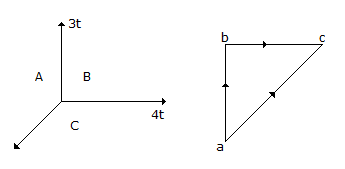# Civil Engineering - UPSC Civil Service Exam Questions

16.

In the force system shown in the figure, the force CA should beA. 5 t B. - 5 t C. 4 t D. - 4 t

Explanation:

No answer description available for this question. Let us discuss.

17.

A cylindrical shell made of mild steel plate of 100 cm diameter is to be subjected to an internal pressure of 10 kg/cm2. If the material yields at 2000 kg/cm2, assuming factor of safety as four and using maximum principal stress theory, thickness of the plate will be

 A. 5 mm B. 10 mm C. 15 mm D. 20 mm

Explanation:

No answer description available for this question. Let us discuss.

18.

A plot of land 60°m x 20 m is measure steel tape. If the standard error of length width measurements is taken as ± 1 cm, the standard error of the area of the plot be

 A. ± 0.1414 m2 B. ± 0.566 m2 C. ± 0.632 m2 D. ± 0.8484 m2

Explanation:

No answer description available for this question. Let us discuss.

19.

The graphical condition for equilibrium of concurrent forces is that

 A. both the force polygon and the funicular polygon must be closed figures B. funicular polygon should be a closed figure C. force polygon need not be a closed figure D. force polygon should be a closed figure

Explanation:

No answer description available for this question. Let us discuss.

20.

In a tilted aerial photograph, if the swing is 230°, then the rotation angle is equal to

 A. 140° B. 130° C. 50° D. 25°